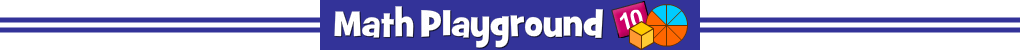AdvertisementParentheses first, exponents next.
Then multiplication and division from left to right.
Follow up with addition and subtraction from left to right.
Quick Calculate - Learning Connections
Essential Skills
Mental Math - practice order of operations

Common Core Connection for Grades 4+
Apply properties of operations as strategies to add and subtract.
Add within 100, including adding a two-digit number and a one-digit number.
Fluently multiply and divide within 100.
Use parentheses, brackets, or braces and evaluate expressions with these symbols.
More Math Games to Play